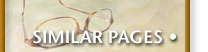## Maps of Maps in Scala

Programmers come in two flavors:

• Those that like iterative operations, like `for`
• Those that prefer set operations, like `map`

Learning from its kitchen-sink-Perl-ancestry, Scala offers both:

``````val nums = Array( 3, 78, 4, 3, 42, 69 )

for( i <- nums if ( i > 10 ) ) yield ( i / 2 )
nums.filter( _ > 10 ).map(_ / 2)
``````

The result of these two statements are exactly the same.

While English is bad enough, when two disciplines come in contact with each other, the progeny from their intercourse isn't always acne-free. In this case, the word map is used in multiple ways.

Consider a "Map" of my wish list††Thank you, Cay Horstmann, for this example, and for your book, Scala for the Impatient :

``````var gizmos = Map( "iPad"->500, "iPhone"->350,
"Thunderbolt" -> 999, "Trackpad" ->49)
``````

Suppose I wanted to show the prices with a 10% discount. You could use the `mapValues()` method, via:

``````gizmos.mapValues( price => price * 0.9 )
``````

With the correct results:

``````Map(iPad -> 450.0, iPhone -> 315.0, Thunderbolt -> 899.1, Trackpad -> 44.1)
``````

But what if I wanted to convert both the key and the value? Yes, we'll use the `.map()` method. However, the following is wrong:

``````gizmos.map( (k,v) => ( "Apple " + k, 0.8 * v ) )
``````

The function we pass in gets a single value as a `Tuple`, which we have to extract.

``````gizmos.map( x => ( "Apple " + x._1, 0.8 * x._2 ) )
``````

Since I'm just learning Scala, I'm sure someone can enlighten me on this, as I would have expected both forms to work.

Tell others about this article: